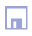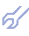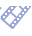In mathematical statistics it is need to have a priori information about the structure of the mathematical model. In neural networks the user estimates this structure by choosing the number of layers and the number and transfer functions of nodes of a neural network. This requires not only knowledge about the theory of neural networks, but also knowledge of the object nature and time. Besides this the knowledge from systems theory about the systems modelled is not applicable without transformation in neural network world. But the rules of translation are unknown. This problems can be overcome by GMDH type neural networks - it can pick out knowledge about object directly from data sampling. The Group Method of Data Handling is the inductive sorting-out method, which has advantages in the cases of rather complex objects, having no definite theory, particularly for the objects with fuzzy characteristics.

The table gives a comparison of both methodologies: neural networks and inductive self-organizing modeling in connection with their application to data analysis.

 Neural networks Statistical learning GMDH networks Data analysis universal approximator universal structure identificator Analytical model indirect approximation direct approximation Architecture preselected unbounded network structure; experimental selection of adequate architecture demands time and experience bounded network structure evolved during estimation process Network synthesis globally optimized fixed network structure adaptively synthesized structure Apriori Information without transformation in the concepts of neural networks not usable can be used directly to select the reference functions and criteria Self-organization deductive, subjective choice of layers number and number of nodes inductive, number of layers and of nodes estimated by minimum of external criterion (objective choice) Parameter estimation in a recursive way; demands long samples estimation on training set by means of maximum likelihood techniques, selection on testing set (may be extremely short or noised) Optimization global search in a highly multimodal space, result depends from initial solution, tedious and requiring from user to set various algorithmic parameters by trial and error, time-consuming technique simultaneously optimize the structure and dependencies in model, not time-consuming technique, inappropriate parameters not included automatically Access to result available transiently in a real-time environment usually stored and repeatedly accessible Initial knowledge needs knowledge about the theory of neural networks necessary knowledge about the kind of task (criterion) and class of system (linear,non-linear) Convergence global convergence is difficult to guarantee model of optimal complexity is founded Computing suitable for implementation using hardware with parallel computation efficient for ordinary computers and also for massively parallel computation Features general-purpose, flexible, non-linear (especially linear) static or dynamic models general-purpose, flexible linear or nonlinear, static or dynamic, parametric and non-parametric models

Results obtained by statistical learning networks and especially GMDH algorithms are comparable with results obtained by neural networks . The well-known problems of an optimal (subjective) choice of the neural network architecture are solved in the GMDH algorithms by means of an adaptive synthesis (objective choice) of the architecture. Such algorithms combining the best features of neural nets and statistical techniques in a powerful way discover the entire model structure directly from data sample - in the form of a network of polynomial functions, difference equations or another structure type. Models are selected automatically based on their ability to solve the task (approximation, identification, forecasting, classification).

Example: Comparison of identification and prediction of the system of equations by ANN and GMDH networks

Let us consider the system of equation

y1t = -2y1t-1 (1 - y1t-1) ;
y2t = 1 + 0.5 y1t-2 y2t-1 ;

where the first is the logistic function. For estimation data with additional noise yit = yit + az was used, where -0.5 < z < 0.5 and a = 0; 0.1; 0.5 .

For a=0 the following model were received by self-organizing algorithm on the basis of 50 observations:

y1t = -2.00 y1t-1 + 2.00 y1t-1 y1t-1 + 2.34e-6,
y2t = 1.002 + 0.50005 y1t-2 y2t-1 + 4.8e-8 y1t-2 ,

Neural networks are unable to identify the system. Several implicit models, distributed in the BP neural network were obtained using 5 input neurons, 2 output and n = 2,4,6,10,20 neurons in the hidden layer. In this example was shown that for large complexity n the approximation error is independent from noise level and models are overfitted. The selected models were used for prediction on 10 steps ahead.

Table: Prediction error MAD = 1/10 * SUM | (y-ym)/y | *100%

 ANN GMDH y1 y2 y1 y2 n a = 0 2 116.0 7.7 0.0 0.0 6 8.0 2.0 10 18.9 1.4 a = 0.1 2 21.1 4.8 6.9 1.3 6 20.6 4.0 10 18.9 3.7 a = 0.5 2 24.6 8.5 29.6 5.0 6 27.0 5.8 10 27.1 10.2

This results were received by Prof.J.-A.Mueller and Dr.F.Lemke. We thank them for help in preparing of this subsection.